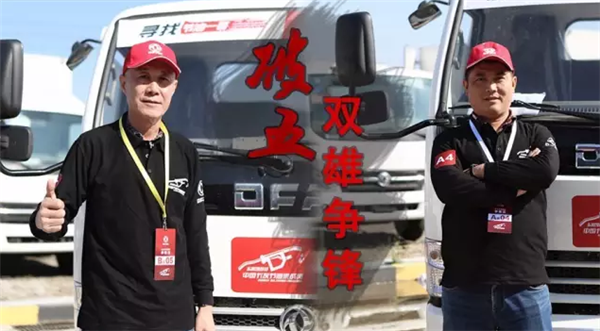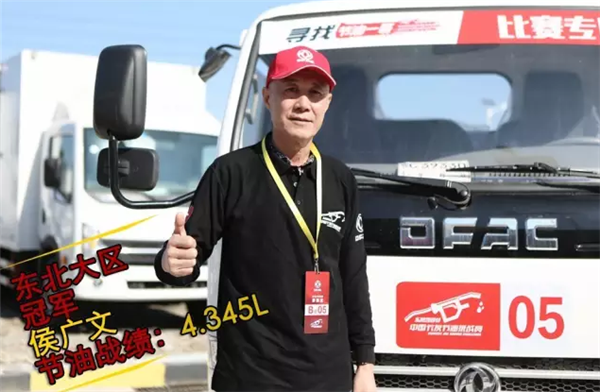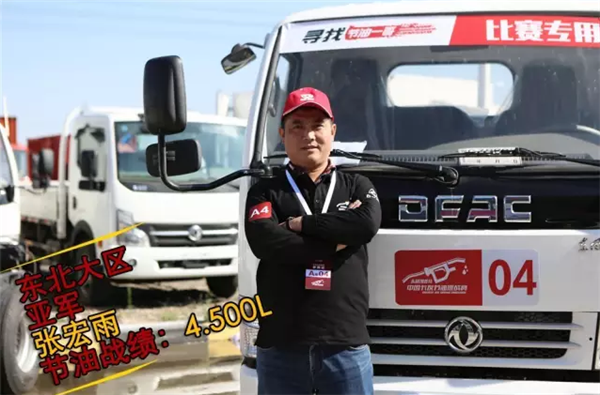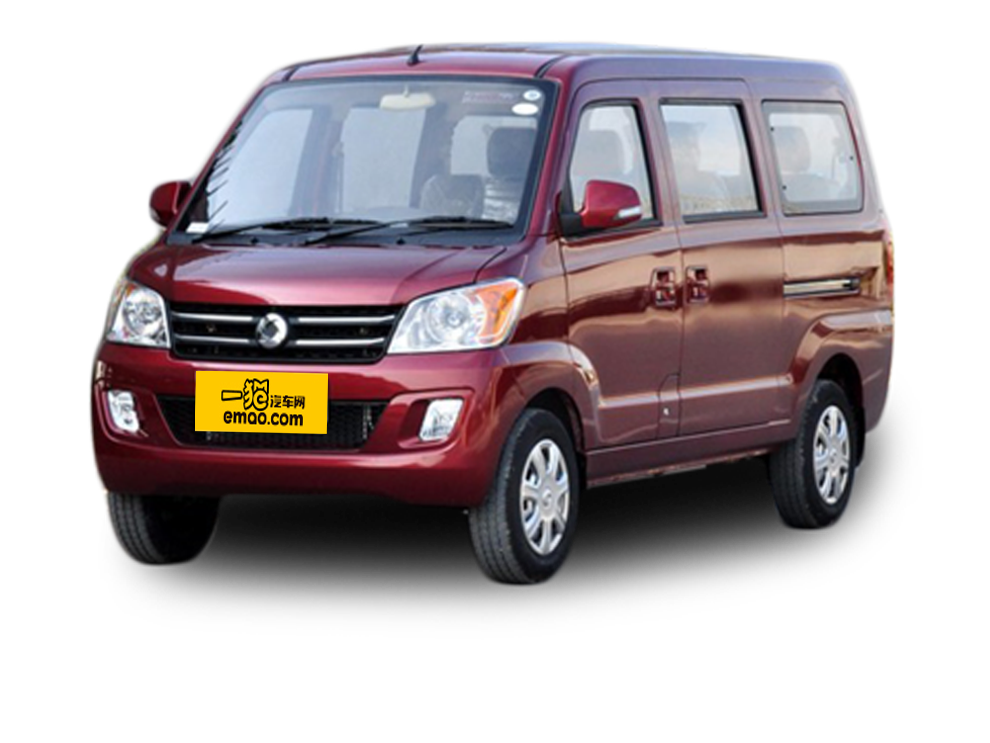【一猫汽车网】近日，东风凯普特·第二届中国卡友节油挑战赛首场复赛在沈阳完美谢幕，从东北7个分赛区突出重围的14位节油精英集聚一堂。高手过招，必有惊喜！来自沈阳本土的侯广文师傅以百公里4.345L油耗的傲人成绩问鼎冠军，夺得东北复赛的头魁；来自哈尔滨赛区的张宏宇也不逊色，以百公里4.50L的成绩获得亚军。冠亚军油耗双双破“五”，为复赛打响一个漂亮的发令枪。姜还是老的辣，这话用在侯广文师傅身上可谓一点也不假。年逾六旬的侯广文是一位在环卫局工作的老师傅，本已到退休年龄的他却割舍不下陪伴自己30余载的卡车。听闻东风有节油赛，侯师傅毫不犹豫就报名参赛，他明白能作为一名卡车司机参赛并证明自己的机会已经不多。侯师傅没有辜负自己，海选时他勇夺单场冠军，复赛又以百公里4.345L的傲人成绩夺得头魁，不得不让人叹服他的老而弥坚。

节油来自点滴，节油源自责任，侯广文师傅的夺冠，决非偶然。“由于我从小见证了大庆油田开发，深知我国石油资源开发的困难，我明白每一滴油都来之不易，我们都应把为国家节省石油资源作为一种光荣使命，并从日常的开车中去点滴践行。”侯广文对节油的认识比一般人都高，他把节油作为一种身体力行的责任。侯师傅所在的环卫局对员工的驾驶技巧和节油驾驶水平都非常重视，每年会有春秋两次的内部节油比赛，30多年驾龄的候师傅通过不断积累养成了非凡的节油驾驶技巧。谈及此，侯广文师傅说，“开好一款车你先要对车的基本状态有一定了解。‘轻离合，慢刹车，缓油门’，注意这三者的配合，只要你用心观察就会有很大的进步。”在比赛开始前的热车阶段，侯师傅先试了试油门反应，对车辆的动力有了一定的了解。在驾驶途中，保持车辆的最佳转速状态，提前预判红绿灯的时间。最终取得超低油耗成绩，确实是多年经验的展现。

在发表获奖感言时候师傅还分享到，要想节油，司机们的经验和技术只是一个方面，更重要的是车辆，车子本身做的好，做的节油，这才是关键，而东风轻卡，就是真正的省油好车。通过比赛，他真正感受了东风轻卡的优异节油性能，以后会给环卫局及身边朋友推荐购买东风轻卡。

从容、淡定、处变不惊，泰山塌于眼前而面不改色，张宏雨凭借沉着冷静的发挥，以每百公里4.500L油耗的好成绩，一举成为东风凯普特·第二届中国卡友节油挑战赛东北赛区复赛的亚军，昂首进军全国总决赛。他的沉稳，与他的经历不无关系。

张宏雨是辽宁辽中人，从16岁开始，张宏雨就参军成为一名光荣的人民解放军战士，进入军队后就一直与车打交道，通过军队的训练开得一手好车。在部队开车期间，他接触了东风品牌的军车，并就此与东风接下了一段不解之缘。退伍后，他利用转业费买了东风轻卡开始从事物流运输。优秀的驾驶技术和职业操守让他的事业发展很快。从2万元资金起步，到现在拥有小有规模的物流公司，张宏雨的创业人生，因为东风更加璀璨精彩。现在张宏雨成立了自己的物流公司，拥有一个由10辆东风车组成的车队，其中有东风多利卡6辆。事业越做越大，生活越过越好，但唯一不变的是十多年来他始终坚持选择东风品牌的信条。现在他的公司业务发展良好，他也有进一步扩大车队的规划，此次前来参加节油大赛，他的目的之一就是想亲身体验、考察东风的新车型。而此次他中意的东风凯普特E280也不负期望，助他油耗“破五”闯进总决赛，成为他下一步考虑购入的目标。

张宏雨的物流公司主要承接哈尔滨地区的优速和韵达这两大快递公司的物流配送业务，平时他也很重视公司车辆的节油问题。“我对公司所有员工提出要求，要提高他们的节油意识和能力。节油首先能直接创造效益，十辆车一年下来，节省下来的油钱可是一笔不菲的数额，再者节油也是在为国家节省能源，构建节约型社会，实现可持续发展，人人有责！”张宏雨说道。作为东风轻卡的忠实用户，他非常认可此次节油赛，希望节油赛能够让更多卡友了解到东风轻卡的实力之余也能提升节能环保意识，为节约型社会献出一份力。

破五，双雄争锋！侯广文和张宏雨凭借着强大的实力晋级全国总决赛，同时也给其它赛区复赛带来了更高的突破标准。作为3000万卡友们平凡的一员，他们却有着不平凡“节油使命感”，他们严格律己，用自己的实际行动证明节油源自点滴，节油源自责任。赛事将继续蔓延，我们有理由期待接下来一场场“真英雄”的较量中涌现出更多超高实力的节油一哥，为我们展现节油技巧的不断精进，节油精神的无限传承。

##### 相关资讯

|分享

•已阅！握爪
•我手滑为你点赞
•128个赞！
•不明觉厉
•阅后既醉
•有钱！任性
•照片太美，我不敢看
•干货！杠杠的
•高大上
•膜拜中！
•请接受我的膝盖
•猫哥/猫妹么么哒
•神吐槽
•我只笑笑不说话
•我想静静
•窒息
##### 资讯相关车系##### 热度排行• 阿斯顿·马丁
• 奥迪
• 阿尔法罗密欧
• ALPINA
• 爱驰
• AC Schnitzer

• 巴博斯
• 宝骏
• 宝马
• 保时捷
• 北汽制造
• 奔驰
• 奔腾
• 本田
• 比亚迪
• 标致
• 别克
• 宾利
• 布加迪
• 北汽威旺
• 北京
• 北汽绅宝
• 北汽幻速
• 北汽新能源
• 宝沃
• 比速汽车
• 北汽道达
• 北京汽车
• 比德文汽车
• 铂驰
• 北汽昌河

• 昌河
• 长安
• 长城
• 长安商用
• 成功汽车
• 长江EV
• 长安轻车型
• 长安欧尚
• 长安凯程
• 长安跨越
• 车驰汽车

• 大众
• 道奇
• 东风
• 东风风神
• 东风小康
• 东南
• DS
• 东风风行
• 东风风度
• 东风风光
• 电咖
• 大乘汽车
• 大运
• 东风富康

• 法拉利
• 菲亚特
• 丰田
• 福迪
• 福特
• 福田汽车
• 福汽启腾
• 风诺
• 福田

• GMC
• 广汽传祺
• 广汽吉奥
• 观致
• 国金汽车
• 广汽新能源
• 广汽集团
• 国机智骏

• 哈飞
• 海格
• 海马
• 华泰
• 黄海
• 恒天
• 红旗
• 哈弗
• 华颂
• 华凯
• 华泰新能源
• 汉腾汽车
• 华利
• 红星汽车
• HYCAN合创
• 汉龙汽车

• Jeep
• 江淮
• 江铃
• 捷豹
• 金杯
• 九龙
• 吉利汽车
• 金旅
• 金龙
• 江铃集团轻汽
• 江铃集团新能源
• 君马汽车
• 捷途
• 钧天
• 捷达
• 几何汽车

• 开瑞
• 凯迪拉克
• 科尼赛克
• 克莱斯勒
• KTM
• 卡威
• 凯翼
• 康迪
• 康迪电动汽车集团
• 开沃汽车
• 卡升

• 猎豹汽车
• 兰博基尼
• 劳斯莱斯
• 雷克萨斯
• 雷诺
• 理念
• 力帆
• 莲花汽车
• 林肯
• 铃木
• 陆风
• 路虎
• 路特斯
• 领志
• 领克
• 零跑汽车
• Lorinser
• LOCAL MOTORS
• LITE
• 领途汽车
• 理想汽车
• 罗夫哈特
• 力帆汽车

• MG
• MINI
• 马自达
• 玛莎拉蒂
• 迈凯伦
• 摩根
• 名爵
• 迈莎锐
• 迈迈

• 纳智捷
• 哪吒汽车

• 讴歌
• 欧宝
• 欧朗
• 欧拉
• 欧尚汽车

• 帕加尼
• Polestar极星

• 奇瑞
• 启辰
• 起亚
• 前途
• 庆铃汽车
• 骐铃汽车
• 全球鹰
• 乔治·巴顿

• 日产
• 荣威
• 瑞麒汽车
• 如虎
• 瑞驰
• 瑞麒
• 瑞驰新能源
• 容大智造

• 上汽大通
• smart
• 三菱
• 双环
• 双龙
• 斯巴鲁
• 斯柯达
• 萨博
• 思铭
• 赛麟
• SWM斯威汽车
• 上汽MAXUS
• SRM鑫源
• 思皓
• SHELBY
• 上喆

• TESLA
• 泰卡特
• 腾势
• 特斯拉
• 天际汽车

• 威麟
• 威兹曼
• 沃尔沃
• 五菱汽车
• 五十铃
• 潍柴英致
• WEY
• 蔚来
• 威马汽车
• 潍柴汽车

• 现代
• 雪佛兰
• 雪铁龙
• 西雅特
• 新特汽车
• 小鹏汽车
• 新宝骏

• 野马汽车
• 一汽
• 依维柯
• 英菲尼迪
• 永源
• 驭胜
• 云度
• 宇通客车
• 云雀汽车
• 远程汽车
• 一汽凌河

• 中华
• 中兴
• 众泰
• 知豆
• 之诺
• 正道汽车
• A
• B
• C
• D
• E
• F
• G
• H
• I
• J
• K
• L
• M
• N
• O
• P
• Q
• R
• S
• T
• U
• V
• W
• X
• Y
• Z

• 阿斯顿·马丁
• 奥迪
• 阿尔法罗密欧
• ALPINA
• 爱驰
• AC Schnitzer

• 巴博斯
• 宝骏
• 宝马
• 保时捷
• 北汽制造
• 奔驰
• 奔腾
• 本田
• 比亚迪
• 标致
• 别克
• 宾利
• 布加迪
• 北汽威旺
• 北京
• 北汽绅宝
• 北汽幻速
• 北汽新能源
• 宝沃
• 比速汽车
• 北汽道达
• 北京汽车
• 比德文汽车
• 铂驰
• 北汽昌河

• 昌河
• 长安
• 长城
• 长安商用
• 成功汽车
• 长江EV
• 长安轻车型
• 长安欧尚
• 长安凯程
• 长安跨越
• 车驰汽车

• 大众
• 道奇
• 东风
• 东风风神
• 东风小康
• 东南
• DS
• 东风风行
• 东风风度
• 东风风光
• 电咖
• 大乘汽车
• 大运
• 东风富康

• 法拉利
• 菲亚特
• 丰田
• 福迪
• 福特
• 福田汽车
• 福汽启腾
• 风诺
• 福田

• GMC
• 广汽传祺
• 广汽吉奥
• 观致
• 国金汽车
• 广汽新能源
• 广汽集团
• 国机智骏

• 哈飞
• 海格
• 海马
• 华泰
• 黄海
• 恒天
• 红旗
• 哈弗
• 华颂
• 华凯
• 华泰新能源
• 汉腾汽车
• 华利
• 红星汽车
• HYCAN合创
• 汉龙汽车

• Jeep
• 江淮
• 江铃
• 捷豹
• 金杯
• 九龙
• 吉利汽车
• 金旅
• 金龙
• 江铃集团轻汽
• 江铃集团新能源
• 君马汽车
• 捷途
• 钧天
• 捷达
• 几何汽车

• 开瑞
• 凯迪拉克
• 科尼赛克
• 克莱斯勒
• KTM
• 卡威
• 凯翼
• 康迪
• 康迪电动汽车集团
• 开沃汽车
• 卡升

• 猎豹汽车
• 兰博基尼
• 劳斯莱斯
• 雷克萨斯
• 雷诺
• 理念
• 力帆
• 莲花汽车
• 林肯
• 铃木
• 陆风
• 路虎
• 路特斯
• 领志
• 领克
• 零跑汽车
• Lorinser
• LOCAL MOTORS
• LITE
• 领途汽车
• 理想汽车
• 罗夫哈特
• 力帆汽车

• MG
• MINI
• 马自达
• 玛莎拉蒂
• 迈凯伦
• 摩根
• 名爵
• 迈莎锐
• 迈迈

• 纳智捷
• 哪吒汽车

• 讴歌
• 欧宝
• 欧朗
• 欧拉
• 欧尚汽车

• 帕加尼
• Polestar极星

• 奇瑞
• 启辰
• 起亚
• 前途
• 庆铃汽车
• 骐铃汽车
• 全球鹰
• 乔治·巴顿

• 日产
• 荣威
• 瑞麒汽车
• 如虎
• 瑞驰
• 瑞麒
• 瑞驰新能源
• 容大智造

• 上汽大通
• smart
• 三菱
• 双环
• 双龙
• 斯巴鲁
• 斯柯达
• 萨博
• 思铭
• 赛麟
• SWM斯威汽车
• 上汽MAXUS
• SRM鑫源
• 思皓
• SHELBY
• 上喆

• TESLA
• 泰卡特
• 腾势
• 特斯拉
• 天际汽车

• 威麟
• 威兹曼
• 沃尔沃
• 五菱汽车
• 五十铃
• 潍柴英致
• WEY
• 蔚来
• 威马汽车
• 潍柴汽车

• 现代
• 雪佛兰
• 雪铁龙
• 西雅特
• 新特汽车
• 小鹏汽车
• 新宝骏

• 野马汽车
• 一汽
• 依维柯
• 英菲尼迪
• 永源
• 驭胜
• 云度
• 宇通客车
• 云雀汽车
• 远程汽车
• 一汽凌河

• 中华
• 中兴
• 众泰
• 知豆
• 之诺
• 正道汽车
• A
• B
• C
• D
• E
• F
• G
• H
• I
• J
• K
• L
• M
• N
• O
• P
• Q
• R
• S
• T
• U
• V
• W
• X
• Y
• Z

• 阿斯顿·马丁
• 奥迪
• 阿尔法罗密欧
• ALPINA
• 爱驰
• AC Schnitzer

• 巴博斯
• 宝骏
• 宝马
• 保时捷
• 北汽制造
• 奔驰
• 奔腾
• 本田
• 比亚迪
• 标致
• 别克
• 宾利
• 布加迪
• 北汽威旺
• 北京
• 北汽绅宝
• 北汽幻速
• 北汽新能源
• 宝沃
• 比速汽车
• 北汽道达
• 北京汽车
• 比德文汽车
• 铂驰
• 北汽昌河

• 昌河
• 长安
• 长城
• 长安商用
• 成功汽车
• 长江EV
• 长安轻车型
• 长安欧尚
• 长安凯程
• 长安跨越
• 车驰汽车

• 大众
• 道奇
• 东风
• 东风风神
• 东风小康
• 东南
• DS
• 东风风行
• 东风风度
• 东风风光
• 电咖
• 大乘汽车
• 大运
• 东风富康

• 法拉利
• 菲亚特
• 丰田
• 福迪
• 福特
• 福田汽车
• 福汽启腾
• 风诺
• 福田

• GMC
• 广汽传祺
• 广汽吉奥
• 观致
• 国金汽车
• 广汽新能源
• 广汽集团
• 国机智骏

• 哈飞
• 海格
• 海马
• 华泰
• 黄海
• 恒天
• 红旗
• 哈弗
• 华颂
• 华凯
• 华泰新能源
• 汉腾汽车
• 华利
• 红星汽车
• HYCAN合创
• 汉龙汽车

• Jeep
• 江淮
• 江铃
• 捷豹
• 金杯
• 九龙
• 吉利汽车
• 金旅
• 金龙
• 江铃集团轻汽
• 江铃集团新能源
• 君马汽车
• 捷途
• 钧天
• 捷达
• 几何汽车

• 开瑞
• 凯迪拉克
• 科尼赛克
• 克莱斯勒
• KTM
• 卡威
• 凯翼
• 康迪
• 康迪电动汽车集团
• 开沃汽车
• 卡升

• 猎豹汽车
• 兰博基尼
• 劳斯莱斯
• 雷克萨斯
• 雷诺
• 理念
• 力帆
• 莲花汽车
• 林肯
• 铃木
• 陆风
• 路虎
• 路特斯
• 领志
• 领克
• 零跑汽车
• Lorinser
• LOCAL MOTORS
• LITE
• 领途汽车
• 理想汽车
• 罗夫哈特
• 力帆汽车

• MG
• MINI
• 马自达
• 玛莎拉蒂
• 迈凯伦
• 摩根
• 名爵
• 迈莎锐
• 迈迈

• 纳智捷
• 哪吒汽车

• 讴歌
• 欧宝
• 欧朗
• 欧拉
• 欧尚汽车

• 帕加尼
• Polestar极星

• 奇瑞
• 启辰
• 起亚
• 前途
• 庆铃汽车
• 骐铃汽车
• 全球鹰
• 乔治·巴顿

• 日产
• 荣威
• 瑞麒汽车
• 如虎
• 瑞驰
• 瑞麒
• 瑞驰新能源
• 容大智造

• 上汽大通
• smart
• 三菱
• 双环
• 双龙
• 斯巴鲁
• 斯柯达
• 萨博
• 思铭
• 赛麟
• SWM斯威汽车
• 上汽MAXUS
• SRM鑫源
• 思皓
• SHELBY
• 上喆

• TESLA
• 泰卡特
• 腾势
• 特斯拉
• 天际汽车

• 威麟
• 威兹曼
• 沃尔沃
• 五菱汽车
• 五十铃
• 潍柴英致
• WEY
• 蔚来
• 威马汽车
• 潍柴汽车

• 现代
• 雪佛兰
• 雪铁龙
• 西雅特
• 新特汽车
• 小鹏汽车
• 新宝骏

• 野马汽车
• 一汽
• 依维柯
• 英菲尼迪
• 永源
• 驭胜
• 云度
• 宇通客车
• 云雀汽车
• 远程汽车
• 一汽凌河

• 中华
• 中兴
• 众泰
• 知豆
• 之诺
• 正道汽车
• A
• B
• C
• D
• E
• F
• G
• H
• I
• J
• K
• L
• M
• N
• O
• P
• Q
• R
• S
• T
• U
• V
• W
• X
• Y
• Z